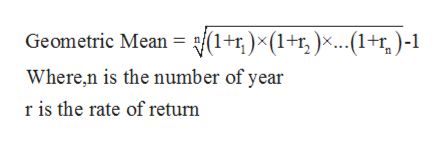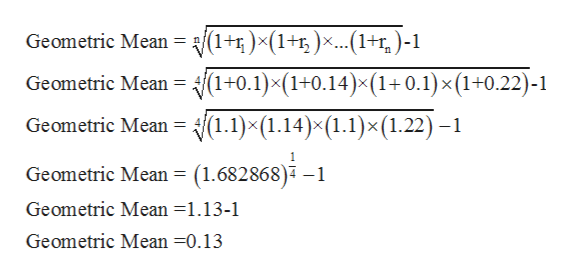# A mutual fund has a rate of return for five years of 10%, 14%, 10%, and 22% . Calculate the geometric mean. Round to the nearest tenth of a percent.

Question
30 views

A mutual fund has a rate of return for five years of 10%, 14%, 10%, and 22% . Calculate the geometric mean. Round to the nearest tenth of a percent.

check_circle

Step 1

Geometric mean: It is the average of a set of observations and is generally used for calculating growth rates like growth rates of population, bonds etc.

The formula for calculating Geometric rate of return is shown above:help_outlineImage Transcriptionclose1+)(1+1, )*..+)-1 Geometric Mean Where,n is the number of year r is the rate of return fullscreen
Step 2

The table showing rate of return for is as follows:

Step 3

The Geometric Mean is calc...help_outlineImage Transcriptionclose|(1+5)x(1+r,)x...(1+r,)-1 Geometric Mean Geometric Mean /(1+0.1)x(1+0.14)x(1+0.1)x(1+0.22)-1 Geometric Mean = H(1.1)x(1.14)x(1.1)x (1.22) -1 Geometric Mean = (1.682868)4 -1 Geometric Mean =1.13-1 Geometric Mean =0.13 fullscreen

### Want to see the full answer?

See Solution

#### Want to see this answer and more?

Solutions are written by subject experts who are available 24/7. Questions are typically answered within 1 hour.*

See Solution
*Response times may vary by subject and question.
Tagged in